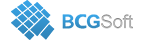BCGSuite for MFC
 LabelContent BCGPChartDataLabelOptions::m_content

Specifies data label content.

Set this option to specify data label content (e.g. what values are taken from a related data point to build the data label text).

It can be one or combination of the following values:

• LC_SERIES_NAME - series name
• LC_CATEGORY_NAME - category name (if series is categorized)
• LC_VALUE - Y value
• LC_PERCENTAGE - percentage (for Pie, Pyramid, Funnel)
• LC_X_VALUE - X value (which can be either a category name or X value for scattered charts)
• LC_BUBBLE_SIZE - bubble size for Bubble series
• LC_BUBBLE_VALUES - all commonly used bubble values: Y value, X value and bubble size
• LC_ALL_BUBBLE_VALUES - all bubble values: series name, Y value, X value, bubble value
• LC_VALUE_PERCENTAGE - value and percentage (for Pie, Pyramid, Funnel)
• LC_SERIES_VALUES - commonly used values: series name, Y value, X value
• LC_SERIES_CATEGORY_VALUE - commonly used values: series name, category name, Y value
• LC_PIE_NAME_PERCENTAGE - commonly used Pie, Pyramid and Funnel values: category name and percentage

Ternary values and percentage:

• LC_A_TERNARY_PERCENTAGE
• LC_B_TERNARY_PERCENTAGE
• LC_C_TERNARY_PERCENTAGE
• LC_A_TERNARY_VALUE
• LC_B_TERNARY_VALUE
• LC_C_TERNARY_VALUE
• LC_ALL_TERNARY_VALUES
• LC_ALL_TERNARY_PERCENTAGE